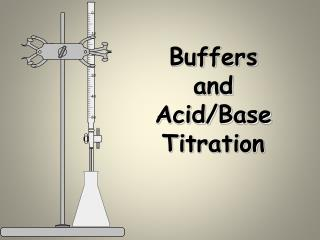DownloadDownload PresentationBuffers and Acid/Base Titration

# Buffers and Acid/Base Titration

Télécharger la présentation## Buffers and Acid/Base Titration

- - - - - - - - - - - - - - - - - - - - - - - - - - - E N D - - - - - - - - - - - - - - - - - - - - - - - - - - -
##### Presentation Transcript

1. Buffers andAcid/Base Titration

2. Common Ion • Suppose we have a solution containing hydrofluoric acid (________) and its salt sodium fluoride (_________). • Major species (HF, Na+, F-, and H2O) • HF slightly dissociated, therefore common ion is _____________ • How does this effect equilibrium of HF dissociation?

3. Common Ion Effect HF(aq)  H+(aq) + F-(aq) Shifts reaction left. Less H+ ions present. Added fluoride ions from NaF • This effect makes a solution of NaF and HF __________ acidic than a solution of HF alone.

4. Buffered Solutions A solution that _____________ a change in pH when either hydroxide ions or protons are added. Buffered solutions contain either: A weak ___________and its salt A weak ___________ and its salt

5. Acid/Salt Buffering Pairs The salt will contain the ___________________ of the acid, and the cation of a strong base (NaOH, KOH)

6. Base/Salt Buffering Pairs The salt will contain the _____________ of the base, and the anion of a strong acid (HCl, HNO3)

7. Henderson-Hasselbalch Equation This is an exceptionally powerful tool, and it’s use will be emphasized in our problem solving.

8. Calculate the pH of the following mixtures: • 0.75 M lactic acid (HC3H5O3) and 0.25 M sodium lactate (Ka = 1.4 x 10-4) • 0.25 M NH3 and 0.40 M NH4Cl (Kb = 1.8 x 10-5)

9. Buffer capacity • The pH of a buffered solution is determined by the ratio ___________________ • As long as this doesn’t change much the pH won’t change much. • The more _________________________these two are the more H+ and OH- the solution will be able to absorb. • Larger concentrations bigger buffer ________________.

10. Buffer Capacity • Calculate the change in pH that occurs when 0.010 mol of HCl(g) is added to 1.0L of each of the following: • 5.00 M HC2H3O2and 5.00 M NaC2H3O2 • 0.050 M HC2H3O2and 0.050 M NaC2H3O2 • Ka= 1.8x10-5

11. Weak Acid/Strong Base Titration A solution that is 0.10 M CH3COOH is titrated with 0.10 M NaOH Equivalence is above pH 7

12. Strong Acid/Strong Base Titration Equivalanceis at pH 7 A solution that is 0.10 M HCl is titrated with 0.10 M NaOH

13. Strong Acid/Strong Base Titration A solution that is 0.10 M NaOH is titrated with 0.10 M HCl Equivalence is at pH 7 It is important to recognize that titration curves are not always increasing from left to right.

14. Strong Acid/Weak Base Titration A solution that is 0.10 M HCl is titrated with 0.10 M NH3 Equivalence is below pH 7

15. Titration of an Unbuffered Solution A solution that is 0.10 M CH3COOH is titrated with 0.10 M NaOH

16. Titration of a Buffered Solution A solution that is 0.10 M CH3COOH and 0.10 M NaCH3COO is titrated with 0.10 M NaOH

17. Comparing Results Buffered Unbuffered

18. Selection of Indicators The equivalence point of a titration, defined by the stoichiometry, is not necessarily the same as the end point, careful selection of the indicator will ensure that the error is ___________________________________.

19. Indicator Transitions Source: Wikipedia

20. Indicators • ____________________________that change color when they become bases. • weak acid written HIn • Weak base • HIn H+ + In- clear red • Equilibrium is controlled by pH • End point - when the indicator changes color.

21. SolubilityEquilibria Lead (II) iodide precipitates when potassium iodide is mixed with lead (II) nitrate. Graphic: Wikimedia Commons user PRHaney

22. Ksp Values for Some Salts at25C

23. Solving Solubility Problems For the salt AgI at 25C, Ksp = 1.5 x 10-16 AgI(s)  Ag+(aq) + I-(aq) 1.5 x 10-16 = x2 x = solubility of AgI in mol/L =

24. Solving Solubility Problems For the salt PbCl2 at 25C, Ksp = 1.6 x 10-5 PbCl2(s)  Pb2+(aq) + 2Cl-(aq) 1.6 x 10-5 = (x)(2x)2 = 4x3 x = solubility of PbCl2 in mol/L =

25. Solving Solubility with a Common Ion For the salt AgI at 25C, Ksp = 1.5 x 10-16 What is its solubility in 0.05 M NaI? AgI(s)  Ag+(aq) + I-(aq) 1.5 x 10-16 = (x)(0.05+x)  (x)(0.05) x = solubility of AgI in mol/L =

26. Precipitation • Ion Product, Q =[M+]a[Nm-]b • If Q>Ksp a precipitate forms. • If Q<Ksp No precipitate. • If Q = Ksp equilibrium.

27. Precipitation A solution of 750.0 mL of 4.00 x 10-3M Ce(NO3)3 is added to 300.0 mL of 2.00 x 10-2M KIO3. Will Ce(IO3)3 (Ksp= 1.9 x 10-10M) precipitate and if so, what is the concentration of the ions?

28. Precipitation and Qualitative Analysis

29. Complex Ions A Complex ion is a charged species composed of: 1. A ____________________________ cation 2.________________________– Lewis bases that have a lone electron pair that can form a covalent bond with an empty orbital belonging to the metallic cation

30. NH3, CN-, and H2O are Common Ligands

31. Coordination Number • Coordination number refers to the number of ligands attached to the ___________________ • ________________________are the most common coordination numbers

32. Complex Ions and Solubility AgCl(s)  Ag+ + Cl- Ksp = 1.6 x 10-10 Ag+ + NH3 Ag(NH3)+ K1 = 2.1 x 103 Ag(NH3)+ NH3 Ag(NH3)2+ K2 = 8.2 x 103 K = KspK1K2 AgCl + 2NH3 Ag(NH3)2+ + Cl-

33. Calculate the concentrations of Ag+, Ag(S2O3)-, and Ag(S2O3)-3 in a solution made by mixing 150.0 mL of 1.00x10-3M AgNO3 with 200.0 mL of 5.00 M Na2S2O3. Ag+ + S2O3-2 Ag(S2O3)- K1=7.4 x 108 Ag(S2O3)- + S2O3-2 Ag(S2O3)-3 K2=3.9 x 104